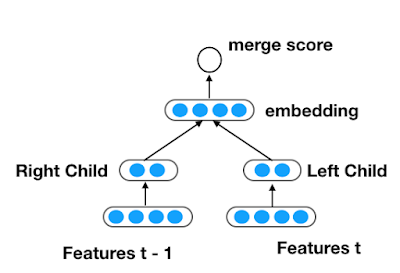## Saturday, September 28, 2019

### Recursive Neural NetworksA Recursive Neural Network

Recursively Neural Networks can learn to structure data such as text or images hierarchically. The network can be seen to work similar to a context free grammar and the output is a parse t
The figure below (from this paper) shows examples of parse trees for an image (top) and a sentence (bottom).

A recursive neural network for sequences always merges two consecutive input vectors and predicts if these two vectors can be merged. If so, we replace the two vectors with best merging score with the hidden vector responsible for the prediction. If done in a recursive manner, we can construct a parse tree.

Formally we can construct the network as follows. Given an input sequence $x = x_1 ... x_T, x_i \in \mathbb{R}^D$ then for two neighboring inputs $x_t, x_{t + 1}$ we predict a hidden representation:

$h_t = \sigma(x_t W_l + b_l)$
$h_{t + 1} = \sigma(x_{t + 1} W_r + b_r)$

The hidden layer used for replacement is computed from the concatenation of the two previous hidden layers: $h=\sigma([h_t, h_{t + 1}] W_h + b_h)$. Finally, the prediction is simply another layer predicting the merging score.

For the implementation we define the neural network with the merging decision first. Given a node with the parent representations (for leaf nodes that are the input vectors) we build the hidden representations and then compute the merging score.
class NeuralGrammar(nn.Module):
'''
Given a tree node we project the left child and right child into
a feature space cl and cr. The concatenated [cl, cr] is projected
into the representation of that node. Furthermore, we predict
the label from the hidden representation.
'''

def __init__(self):
super(NeuralGrammar, self).__init__()
self.left       = nn.Linear(IN, H, bias=True)
self.right      = nn.Linear(IN, H, bias=True)
self.parent     = nn.Linear(2 * H, IN, bias=True)
self.projection = nn.Linear(IN, 1, bias=True)

def forward(self, node):
l = node.left_child.representation
r = node.right_child.representation
y = node.label
x = torch.cat([torch.relu(self.left(l)), torch.relu(self.right(r))], 0)
p = torch.tanh(self.parent(x))
score = self.projection(p)
return (p, score)


Now we can implement our node class which also handles the parsing, by greedily merging up to a tree. A node in the tree holds it's representation as well as a left and a right child. In other words, each node represents a merging decision with the two children being the nodes merged and the parent representation being the hidden layer in the merger grammar. Parsing a tree involves merging the vectors with the highest score and replacing the nodes with their parent node.

class TreeNode:
'''
A tree node in a neural grammar.
Represented as a binary tree. Each node is also represented by
an embedding vector.
'''

def __init__(self, representation=None):
self.representation = representation
self.left_child     = None
self.right_child    = None

def greedy_tree(cls, x, grammar):
'''
Greedily merges bottom up using a feature extractor and grammar
'''
T, D   = x.shape
leafs  = [cls(x[i, :]) for i in range(T)]
while len(leafs) >= 2:
max_score       = float('-inf')
max_hypothesis  = None
max_idx         = None
for i in range(1, len(leafs)):
# difference of reconstruction of the center of the span of the subtree in the spectrogram is the score
# inspired by continuous bag of words
hypothesis = cls(leafs[i - 1].start, leafs[i].stop)
hypothesis.left_child     = leafs[i - 1]
hypothesis.right_child    = leafs[i]
representation, score     = grammar(hypothesis)
hypothesis.representation = representation
if score > max_score:
max_score      = score
max_hypothesis = hypothesis
max_idx        = i
leafs[max_idx - 1:max_idx + 1] = [max_hypothesis]
return leafs


During learning, we collect the scores of the subtrees and use labels to decide if a merge was correct or not.